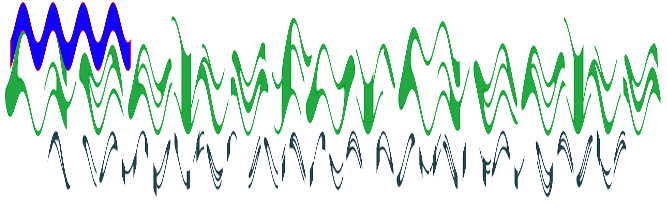Open in App
Not now

# Node.js GM wave() Function

• Last Updated : 13 Oct, 2021

The wave() function is an inbuilt function in the GraphicsMagick library which is used to alter an image along with a sine wave. The function returns the true value on success.

Syntax:

`wave( amplitude, wavelength )`

Parameters: This function accept two parameters as mentioned above and described below:

• amplitude: This parameter stores the value of the amplitude of the sine wave.
• wavelength: This parameter stores the value of the wavelength of the sine wave.

Return Value: This function returns GraphicsMagick object with image added.

Original Image:Example 1:

 `// Include gm library ` `var` `gm = require(``'gm'``); ` ` `  `// Import the image ` `gm(``'gfg.png'``) ` ` `  `// Invoke wave function with an amplitude ` `// values as 10 and wavelength as 3 ` `.wave(10, 3) ` ` `  `// Process and Write the image ` `.write(``"wave1.png"``, ``function` `(err) { ` `  ``if` `(!err) console.log(``'done'``); ` `}); `

Output:Example 2:

 `// Include gm library ` `var` `gm = require(``'gm'``); ` `  `  `// Import the image ` `gm(``'gfg.png'``) ` `  `  `// Set stroke color ` `.stroke(``"#fe1232"``) ` `  `  `// Set fill color ` `.fill(``"#1200ff"``) ` `  `  `// Draw Rectangle using drawRectangle function ` `.drawRectangle(10, 2, 130, 30, 1, 2) ` `  `  `// Invoke wave function with an amplitude ` `// as 20 and wavelength as 30 ` `.wave(20, 30) ` `  `  `// Process and Write the image ` `.write(``"wave2.png"``, ``function` `(err) { ` `    ``if` `(!err) console.log(``'done'``); ` `}); `

Output:Reference:

My Personal Notes arrow_drop_up
Related Articles